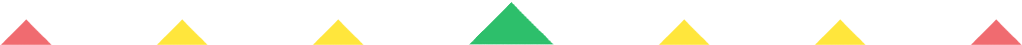Player 1
vs
Player 2
0:00
Opponent history
x
xCorrect
0
Wrong
0
Correct!
Wrong!
Help Sheet for

• ? + 0 = 0
• ? + 0 = 1
• ? + 0 = 2
• ? + 0 = 3
• ? + 0 = 4
• ? + 0 = 5
• ? + 0 = 6
• ? + 0 = 7
• ? + 0 = 8
• ? + 0 = 9
• ? + 0 = 10
• ? + 0 = 11
• ? + 0 = 12

• ? + 1 = 2
• ? + 1 = 3
• ? + 1 = 4
• ? + 1 = 5
• ? + 1 = 6
• ? + 1 = 7
• ? + 1 = 8
• ? + 1 = 9
• ? + 1 = 10
• ? + 1 = 11
• ? + 1 = 12
• ? + 1 = 13

• ? + 2 = 4
• ? + 2 = 5
• ? + 2 = 6
• ? + 2 = 7
• ? + 2 = 8
• ? + 2 = 9
• ? + 2 = 10
• ? + 2 = 11
• ? + 2 = 12
• ? + 2 = 13
• ? + 2 = 14

• ? + 3 = 6
• ? + 3 = 7
• ? + 3 = 8
• ? + 3 = 9
• ? + 3 = 10
• ? + 3 = 11
• ? + 3 = 12
• ? + 3 = 13
• ? + 3 = 14
• ? + 3 = 15

• ? + 4 = 8
• ? + 4 = 9
• ? + 4 = 10
• ? + 4 = 11
• ? + 4 = 12
• ? + 4 = 13
• ? + 4 = 14
• ? + 4 = 15
• ? + 4 = 16

• ? + 5 = 10
• ? + 5 = 11
• ? + 5 = 12
• ? + 5 = 13
• ? + 5 = 14
• ? + 5 = 15
• ? + 5 = 16
• ? + 5 = 17